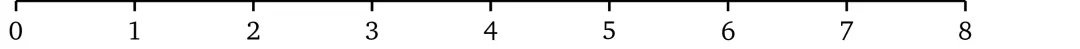# 从零开始学习zk-SNARK(一)-多项式的性质与证明

https://www.interchains.cc/22463.html

### 从零开始学习zk-SNARK(一)-多项式的性质与证明

• zk-SNARK

17 年最早接触 zk-SNARK 开始，就断断续续得学习了一些 zk-SNARK 的知识，但对其原理始终存在诸多困惑，没有形成一个完整的认识。偶然一次机会，看到了 Maksym Petkus 的这篇文章。文章从最基本的多项式性质讲起，从一个简单易懂的证明协议开始，然后像堆积木一样在发现问题，修改问题中逐步去完善协议，直到最终构造出完整的 zk-SNARK 协议。另外作者这种从问题出发的讲解方式，让读者知其然，也知其所以然 。作为一枚毕业多年早把数学知识还给老师的程序媛，读到这篇文章如获至宝，这篇文章读下来的感受像找到了一个脚手架，将脑海里碎片化的知识逐渐拼凑完整。于是想把它翻译出来（已获得作者授权），一方面加深自己的学习，另一方面也将这份宝藏分享给小伙伴们。 文章翻译存在不足之处，欢迎纠正，补充，指导。 —— [email protected]安比实验室

【本系列文章】

1. 多项式的性质与证明
2. 多项式的非交互式零知识证明
3. 从程序到多项式的构造
4. 多项式的约束
5. Pinocchio 协议

## 序言和介绍

Zero-knowledge succinct non-interactive arguments of knowledge (zk-SNARK) 确实是一种非常精妙的方法，它可以在不揭示任何信息的前提下证明某个论断为真。但首要问题是，它为什么有用？

1. 证明关于隐私数据的声明：

• 一个人 A 的银行账户金额多于 X
• 去年，一家银行未与实体 Y 进行交易
• 在不暴露全部 DNA 数据的前提下匹配 DNA
• 一个人的信用评分高于 Z
2. 匿名认证：

• 在不揭露身份的情况下（比如登录密码），证明请求者 R 有权访问网站的受限区域
• 证明一个人来自一组被允许的国家/地区列表中的某个国家/地区，但不暴露具体是哪个
• 证明一个人持有地铁月票，而不透露卡号
3. 匿名支付：

• 付款完全脱离任何一种身份
• 纳税而不透露收入
4. 外包计算

• 将昂贵的计算外包，并在不重新执行的情况下验证结果是否正确；它打开了一种零信任计算的类别
• 改进区块链blockchain模型，从所有节点做同样的计算，到只需一方计算然后其它节点进行验证

• 完整性 —— 只要「陈述」是正确的，prover 就可以让 verifier 确信
• 可靠性 —— 如果「陈述」是错误的，那么作弊的 prover 就没有办法让 verifier 相信
• 零知识 —— 协议的交互仅仅揭露「陈述」是否正确而不泄漏任何其它的信息

zk-SNARK 这个术语本身是在 [Bit+11]中引入的，它在[Gro10]的基础上，又遵循了匹诺曹协议[Gen+12]; [Par+13]使其能够适用于通用的计算。

## 证明的媒介

verifier 一次只能检查（即，读）一位。为了验证这个陈述，我们以某种任意的顺序读取元素并检查其是否确实等于 1 。如果第一次抽样检查的结果是 1，就设置「陈述」的可信度为 ⅒= 10％，否则，如果等于 0，就说明「陈述」是错误的。验证者继续进行下一轮验证，直到获得足够的可信度为止。假如在一些场景下要信任 prover 需要至少 50% 的可信度，那就意味着必须执行 5 次校验。但假如在其它一些场景下需要 95% 的可信度，就需要检查所有的元素。很明显这个证明协议的缺点是必须要根据元素的数量进行检查，如果我们处理数百万个元素的数组，这么做是不现实的。

x³ – 6x² + 11x – 6 =x³ – 6x² + 10x – 5 x-1=0

x³ – 6x² +11x – 6 = 504 x³ – 6x² +10x – 5 = 495

• verifier 选择一个随机值 x 并在本地计算多项式结果
• verifier 将 x 值给到 prover，并让他计算相关的多项式结果
• prover 代入 x 到多项式计算并将结果给到 verifier
• verifier 检查本地的计算结果和 prover 的计算结果是否相等，如果相等那就说明 prover 的陈述具有较高的可信度

@Maksym（作者） 与低效的位检查协议相比，新的协议只需要一轮验证就可以让陈述具有非常高的可信度（假设 d 远小于 x 取值范围的上限，就几乎是 100%了）

## 证明多项式的知识

\$ c_nx^n+ .. +c_1x^1+c_0x^0 \$

## 因式分解

\$(x-a_0)(x-a_1) ..(x-a_n)=0\$

\$x^3 – 3x^2 + 2x =(x-0)(x-1)(x-2)\$

(x-1)(x-2) · …

p(x) = t(x) · h(x)

\$ h(x)=frac{p(x)}{t(x)} \$

• verifier 挑选一个随机值 r, 计算 t = t(r) (即，求值) ，然后将 r 发送给 prover。
• prover 计算 h(x) =p(x) / t(x) ，并对 p(r) 和 h(r) 进行求值，将计算结果 p, h 提供给 verifier。
• verifier 验证 p= t⋅h，如果多项式相等，就意味着 t(x) 是 p(x) 的因式。

• verifier 选一个随机数 23，并计算 t = t(23) = (23 – 1)(23 – 2) = 462，然后将 23 发给 prover
• prover 计算 h(x) =p(x) / t(x) = x, 并对 p(r) 和 h(r) 进行求值，p= p(23) = 10626，h= h(23) = 23，将 p 和 h 提供给 verifier
• verifier 再验证 p= t⋅h：10626 = 462 ⋅ 23 是正确的，这样陈述就被证明了。

@Maksym（作者） 虽然为了简化而使用了一组数学符号，但是如果忽视这个无处不在的基本符号：’(上撇)的话将不利于理解。这个符号本质目的是为了强调一个经过初始变量变换或者推导得到的新变量。即，如果我们想要将 v 乘以 2 并给将它赋值给一个新的变量，我们可以使用：v’= 2 ⋅ v。

\$h(x)=2x+3+frac{7x-6}{t(x)}\$ 。

Remark 3.1现在我们就可以在不知道多项式的前提下根据特定的性质来验证多项式了，这就已经给了我们一些零知识和简明性的特性。但是，这个结构中还存在好多问题：

• prover 可能并不知道他所声称的 p(x)，他可以先算一下 t = t(r)，然后选择一个随机值 h，由此计算出 p = t⋅h。因为等式是成立的，所以也能通过 verifier 的校验。
• 因为 prover 知道随机点 x = r ，他可以构造出一个任意的多项式，这个任意多项式与 t(r) ⋅ h(r) 在 r 处有共同点。
• 在前面的「陈述」中，prover 声称他知道一个特定阶数的多项式，但现在的协议对阶数并没有明确的要求。因而 prover 完全可以拿一个满足因式校验的更高阶数的多项式来欺骗 verifier。

1. prover 知道了 t(r)，他就可以反过来任意构造一个可以整除 t(r) 的 p(r)
2. prover 知道了点(r,t(r) · h(r)) 的值，就可以构造经过这一点的任意多项式，同样满足校验
3. 协议并没有对 prover 的多项式阶数进行约束

## 模糊计算

Remark 3.1 中的前两个问题是由于 暴露了原始值 而导致的，也就是 prover 知道了 r 和 t(r)。但如果 verifier 给出的这个值像放在黑盒里一样不可见的话就完美了，也就是一个人即使不破坏协议，也依然能在这些模糊的值上面完成计算。有点类似哈希函数，从计算结果就很难再回到原始值上。

### 模运算3 × 5 = 3 mod 6 5 + 2 = 1 mod 6

2 × 4 = 1 mod 6 2 – 1 = 1 mod 6 1 × 3 = 3 mod 6

5 × 4 = 2 mod 6 4 × 2 = 2 mod 6 2 × 1 = 1 mod 6 ……

### 强同态加密

5<sup>1</sup> = 5(mod 7)

5<sup>2</sup> = 4(mod 7)

5<sup>3</sup> = 6(mod 7)

……

5<sup>11</sup> = 3(mod 7)

5<sup>17</sup> = 3(mod 7) …… 这样就很难知道指数是多少了。事实上，如果模取得相当大，从运算结果倒推指数运算就不可行了；现代密码学很大程度上就是基于这个问题的“困难”。

encryption: 5<sup>3</sup> = 6 (mod 7)

multiplication: 6<sup>2</sup> = (5<sup>3</sup>)<sup>2</sup> = 5<sup>6</sup>= 1 (mod 7)

addition: 5<sup>3</sup> · 5<sup>2</sup> = 5<sup>5</sup> = 3(mod 7)

\$E(v) = g^v (mod n)\$

Remark 3.2 这个同态加密模式有一个限制，我们可以把一个加密的值和一个未加密的值相乘，但我们不能将两个加密的值相乘（或者相除），也就是说我们不能对加密值取幂。虽然这些性质第一感觉看起来很不友好，但是这却构成了 zk-SNARK 的基础。这个限制后面将在“加密值乘法”一节中讲到。

### 加密多项式

\$E {(x^3)}^1cdot E {(x^2)}^{-3} cdot E {(x)}^2 = {({g^x}^3)}^1 cdot {({g^x}^2)}^{-3} cdot {(g^x)}^2 = g^{1x^3} cdot (g^{-3x^2}) cdot {g^x}^2 = g^{x^3-3x^2+2x}\$

• Verifier

• 取一个随机数 s ，也就是秘密值

• 指数 i 取值为 0，1，…，d 时分别计算出 s 的 i 次幂的加密结果，即：\$E{(s^i)}=g^i\$

• 代入 s 计算未加密的目标多项式：\$t(s)\$

• 将对 s 的幂的加密值提供给 prover:\$E{(s^0)},E{(s^1)},..,E{(s^d)}\$

• Prover

• 计算多项式 h(x) = p(x)/t(x)

• 使用加密值\${g^s}^0,{g^s}^1,..,{g^s}^d,\$\$和系数 \$\$ c_0,c_1,..,c_n \$计算

• 然后同样计算\$ E(h(s)) =g^h(s)\$

• 将结果 \$g^p\$ 和 \$g^h\$ 提供给 verifier

• Verifier

• 最后一步是 verifier 去校验

\$p=t(s) cdot h:g^p ={(g^h)}^{t(s)}=>g^p=g^{t(s)cdot h}\$

### reference

[Bit+11] — Nir Bitansky, Ran Canetti, Alessandro Chiesa, and Eran Tromer. From Extractable Collision Resistance to Succinct Non-Interactive Arguments of Knowledge, and Back Again. Cryptology ePrint Archive, Report 2011/443. https://eprint.iacr.org/2011/443. 2011

[Par+13] — Bryan Parno, Craig Gentry, Jon Howell, and Mariana Raykova. Pinocchio: Nearly Practical Verifiable Computation. Cryptology ePrint Archive, Report 2013/279. https://eprint.iacr.org/2013/279. 2013

[Rei16] — Christian Reitwiessner. zkSNARKs in a Nutshell. 2016. url: https://blog.ethereum.org/2016/12/05/zksnarks-in-a-nutshell/

[But16] — Vitalik Buterin. Quadratic Arithmetic Programs: from Zero to Hero. 2016. url: https://medium.com/@VitalikButerin/quadratic-arithmetic-programs-from-zero-to-hero-f6d558cea649

[But17] — Vitalik Buterin. zk-SNARKs: Under the Hood. 2017. url: https://medium.com/@VitalikButerin/zk-snarks-under-the-hood-b33151a013f6

[Gab17] — Ariel Gabizon. Explaining SNARKs. 2017. url: https://z.cash/blog/snark-explain/

[Ben+14] — Eli Ben-Sasson, Alessandro Chiesa, Christina Garman, Matthew Green, Ian Miers, Eran Tromer, and Madars Virza. Zerocash: Decentralized Anonymous Payments from Bitcoin. Cryptology ePrint Archive, Report 2014/349. https://eprint.iacr.org/2014/349. 2014

[GMR85] — S Goldwasser, S Micali, and C Rackoff. “The Knowledge Complexity of Interactive Proof-systems”. In: Proceedings of the Seventeenth Annual ACM Symposium on Theory of Computing. STOC ’85. Providence, Rhode Island, USA: ACM, 1985, pp. 291–304. isbn: 0–89791–151–2. doi: 10.1145/22145.22178. url: http://doi.acm.org/10.1145/22145.22178

[BFM88] — Manuel Blum, Paul Feldman, and Silvio Micali. “Non-interactive Zero-knowledge and Its Applications”. In: Proceedings of the Twentieth Annual ACM Symposium on Theory of Computing. STOC ’88. Chicago, Illinois, USA: ACM, 1988, pp. 103–112. isbn: 0–89791–264–0. doi: 10.1145/62212.62222. url: http://doi.acm.org/10.1145/62212.62222

[Gro10] — Jens Groth. “Short pairing-based non-interactive zero-knowledge arguments”. In: International Conference on the Theory and Application of Cryptology and Information Security. Springer. 2010, pp. 321–340

[Gen+12] — Rosario Gennaro, Craig Gentry, Bryan Parno, and Mariana Raykova. Quadratic Span Programs and Succinct NIZKs without PCPs. Cryptology ePrint Archive, Report 2012/215. https://eprint.iacr.org/2012/215. 2012

[Pik13] — Scott Pike. Evaluating Polynomial Functions. 2013. url: http://www.mesacc.edu/~scotz47781/mat120/notes/polynomials/evaluating/evaluating.html

[Pik14] — Scott Pike. Dividing by a Polynomial. 2014. url: http://www.mesacc.edu/~scotz47781/mat120/notes/divide_poly/long_division/long_division.html